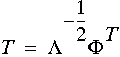• Linear transformation for data in new space is computed usingThe main objective of transformation is to make covariance matrix of Y an identity matrix. We desire T to be orthonormal transformation.

• Ideal transform for new space is given bywhereare the eigenvalues andare the eigenvectors.